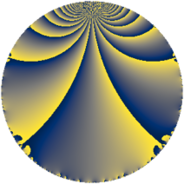# Properties

 Label 99.3.iLevel $99$ Weight $3$ Character orbit 99.i Rep. character $\chi_{99}(23,\cdot)$ Character field $\Q(\zeta_{6})$ Dimension $40$ Newform subspaces $1$ Sturm bound $36$ Trace bound $0$

# Related objects

## Defining parameters

 Level: $$N$$ $$=$$ $$99 = 3^{2} \cdot 11$$ Weight: $$k$$ $$=$$ $$3$$ Character orbit: $$[\chi]$$ $$=$$ 99.i (of order $$6$$ and degree $$2$$) Character conductor: $$\operatorname{cond}(\chi)$$ $$=$$ $$9$$ Character field: $$\Q(\zeta_{6})$$ Newform subspaces: $$1$$ Sturm bound: $$36$$ Trace bound: $$0$$

## Dimensions

The following table gives the dimensions of various subspaces of $$M_{3}(99, [\chi])$$.

Total New Old
Modular forms 52 40 12
Cusp forms 44 40 4
Eisenstein series 8 0 8

## Trace form

 $$40 q - q^{3} + 40 q^{4} - 9 q^{5} - 22 q^{6} + 2 q^{7} - 11 q^{9} + O(q^{10})$$ $$40 q - q^{3} + 40 q^{4} - 9 q^{5} - 22 q^{6} + 2 q^{7} - 11 q^{9} - 62 q^{12} - 10 q^{13} - 18 q^{14} - 40 q^{15} - 80 q^{16} + 4 q^{18} - 28 q^{19} + 54 q^{20} - 4 q^{21} + 144 q^{23} + 114 q^{24} + 97 q^{25} - 130 q^{27} + 32 q^{28} - 216 q^{29} + 2 q^{30} + 47 q^{31} + 36 q^{32} - 22 q^{33} + 12 q^{34} + 74 q^{36} + 86 q^{37} + 90 q^{38} + 32 q^{39} - 60 q^{40} - 216 q^{41} + 158 q^{42} - 64 q^{43} + 373 q^{45} - 216 q^{46} + 18 q^{47} + 214 q^{48} - 162 q^{49} - 522 q^{50} - 46 q^{51} + 62 q^{52} - 112 q^{54} - 66 q^{55} + 72 q^{56} + 150 q^{57} + 90 q^{58} - 387 q^{59} - 314 q^{60} + 110 q^{61} - 92 q^{63} + 196 q^{64} - 306 q^{65} + 110 q^{66} - 109 q^{67} + 882 q^{68} + 181 q^{69} - 120 q^{70} + 36 q^{72} - 196 q^{73} + 54 q^{74} - 5 q^{75} + 44 q^{76} - 394 q^{78} - 34 q^{79} + 469 q^{81} - 300 q^{82} + 792 q^{83} - 650 q^{84} + 96 q^{85} + 720 q^{86} + 708 q^{87} - 1124 q^{90} + 52 q^{91} + 414 q^{92} + 311 q^{93} - 66 q^{94} - 144 q^{95} + 484 q^{96} - 103 q^{97} + 55 q^{99} + O(q^{100})$$

## Decomposition of $$S_{3}^{\mathrm{new}}(99, [\chi])$$ into newform subspaces

Label Dim $A$ Field CM Traces $q$-expansion
$a_{2}$ $a_{3}$ $a_{5}$ $a_{7}$
99.3.i.a $40$ $2.698$ None $$0$$ $$-1$$ $$-9$$ $$2$$

## Decomposition of $$S_{3}^{\mathrm{old}}(99, [\chi])$$ into lower level spaces

$$S_{3}^{\mathrm{old}}(99, [\chi]) \cong$$ $$S_{3}^{\mathrm{new}}(9, [\chi])$$$$^{\oplus 2}$$